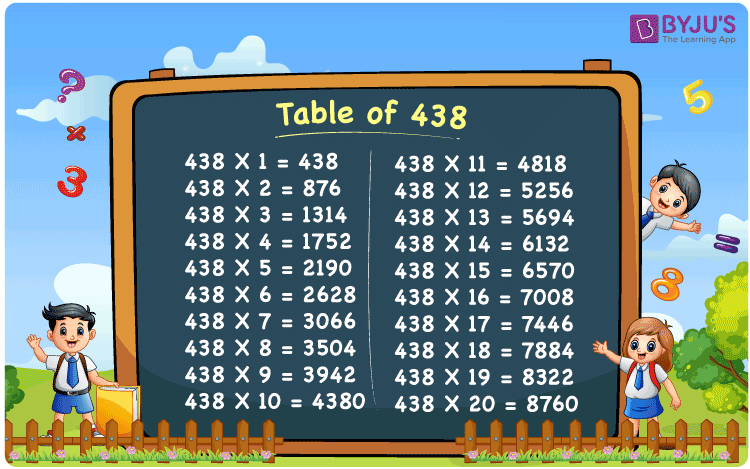Checkout JEE MAINS 2022 Question Paper Analysis : Checkout JEE MAINS 2022 Question Paper Analysis :

# Table of 438

The Table of 438 is beneficial to students who aspire to do well in their final exam. 438 + 438 = 876 is the result of multiplying 438 by two. 438  x 2 equals 876, according to the tables. This is where you will find the multiplication of 438 up to 20. Students who are working on Math problems can use the multiplication table as a reference. From the link provided below, you can download and print the PDF of the table of 438 for free.

## Table of 438 Chart## What is the 438 Times Table?

When we multiply 438 with natural numbers, the table of 438 helps us understand the addition of 438. Children will gain a strong understanding of multiplication by memorising the table 438, and it will also be important in their future studies.

 438×1 = 438 438 438×2 = 876 438 + 438 = 876 438×3 = 1314 438 + 438 + 438 = 1314 438×4 = 1752 438 + 438 + 438 + 438 = 1752 438×5 = 2190 438 + 438 + 438 + 438 + 438 = 2190 438×6 = 2628 438 + 438 + 438 + 438 + 438 + 438 = 2628 438×7 = 3066 438 + 438 + 438 + 438 + 438 + 438 + 438 = 3066 438×8 = 3504 438 + 438 + 438 + 438 + 438 + 438 + 438 + 438 = 3504 438×9 = 3942 438 + 438 + 438 + 438 + 438 + 438 + 438 + 438 + 438 = 3942 438×10 = 4380 438 + 438 + 438 + 438 + 438 + 438 + 438 + 438 + 438 + 438 = 4380

## Multiplication Table of 438

The first 20 multiples of 438 are shown in the multiplication table below.

 438 × 1 = 438 438 × 2 = 876 438 × 3 = 1314 438 × 4 = 1752 438 × 5 = 2190 438 × 6 = 2628 438 × 7 = 3066 438 × 8 = 3504 438 × 9 = 3942 438 × 10 = 4380 438 × 11 = 4818 438 × 12 = 5256 438 × 13 = 5694 438 × 14 = 6132 438 × 15 = 6570 438 × 16 = 7008 438 × 17 = 7446 438 × 18 = 7884 438 × 19 = 8322 438 × 20 = 8760

## Solved Example on the Table of 438

Q. Calculate 438 x 15 + 10.

Solution: Given,

438 x 15 + 10

= 6570 + 10

= 6580

## Frequently Asked Questions on the Table of 438

### How is the table of 438 useful for students?

The table of 438 has multiplication of 438 up to 20 in the table format. By using this as a reference, students will be able to solve various problems, both at the primary and secondary level.

### Using the table, find 438 times 5.

The value of 438 times 5 is 438 × 5 = 2190.

### Calculate 438 multiplied by 10.

438 multiplied by 10 is 4380. In the table of 438,
438 x 10 = 4380